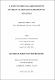## A rapid method for approximating invariant manifolds of differential equations2006
##### Authors
Tang, Shouchun (Terry)
University of Lethbridge. Faculty of Arts and Science
##### Publisher
Lethbridge, Alta. : University of Lethbridge, Faculty of Arts and Science, 2006
##### Abstract
The Intrinsic Low-Dimensional Manifold (ILDM) has been adopted as an approximation to the slow manifold representing the long-term evolution of a non-linear chemical system. The computation of the slow manifold simplifies the model without sacrificing accuracy because the trajectories are rapidly attracted to it. The ILDM has been shown to be a highly accurate approximation to the manifold when the curvature of the manifold is not too large. An efficient method of calculating an approximation to the slow manifold which may be equivalent to the ILDM is presented. This method, called Functional Equation Truncation (FET). is based on the assumption that the local curvature of the manifold is negligible, resulting in a locally linearized system. This system takes the form of a set of algebraic equations which can be solved for given values of the independent variables. Two-dimensional and three-dimensional models are used to test this method. The approximations to onedimensional slow manifolds computed by FET are quite close to the corresponding ILDMs and those for two-dimensional ones seem to differ from their ILDM counterparts.
##### Description
vii, 61 leaves ; 29 cm.
##### Keywords
Dissertations, Academic , Manifolds (Mathematics) , Low-dimensional topology , Differential equations